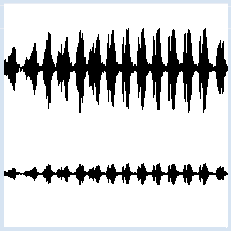deutsch     english    français     Print

## 4.2 SOUND EDITING### INTRODUCTION

 As you know, sound samples (sampling values of a sound) are stored in a list and can be played back again with this list. If you want to edit the sound, you can easily change the list accordingly. PROGRAMMING CONCEPTS: Rectangular wave, integer division, modulo operation

### CHANGING THE LOUDNESS/VOLUME

 The program should reduce the volume of the sound to one quarter. To do this, copy the sound list to another list, where each list element is set to ¼ of its original value. ```from soundsystem import * samples = getWavMono("mysound.wav") soundlist = [] for item in samples: soundlist.append(item // 4) openMonoPlayer(soundlist, 22010) play() ``` Highlight program code (Ctrl+C copy, Ctrl+V paste)

### MEMO

 To copy a list, first create an empty list then fill it using append(). In order to get a list of integers again, you need to use integer division (double division slash).

### USING THE LIST INDEX

 In the following example, you go through the list using the list index and change the list elements without creating a new list. Display the sound graphically before and after the change.```from soundsystem import *
from gpanel import *

samples = getWavMono("mysound.wav")

makeGPanel(0, len(samples), -33000, 33000)
for i in range(len(samples)):
if i == 0:
move(i, samples[i] + 10000)
else:
draw(i, samples[i] + 10000)

for i in range(len(samples)):
samples[i] = samples[i] // 4

for i in range(len(samples)):
if i == 0:
move(i, samples[i] - 10000)
else:
draw(i, samples[i] - 10000)
```
Highlight program code (Ctrl+C copy, Ctrl+V paste)

### MEMO

 People often use the variable name i as a list index. If you run through a loop block using a for structure for i in range(10): i is also called a stepper.

### GENERATING SOUNDS

 It is exciting to create your own sounds, not by loading a sound list from a sound file, but rather by creating the list elements yourself. To make a "rectangular wave sound" you repeatedly store  the value 5000 in the list, for a certain index range, and subsequently -5000 for the same index range. ```from soundsystem import * samples = [] for i in range(4 * 5000): value = 5000 if i % 10 == 0: value = -value samples.append(value) openMonoPlayer(samples, 5000) play() ``` Highlight program code (Ctrl+C copy, Ctrl+V paste)

### MEMO

 The sampling rate of 10000 Hz corresponds to a sound sample every 0.1 ms. We want to change the sign (-/+) always after 10 values, in other words, every 1 ms. This corresponds to a rectangular wave period of 2 ms, which yields a sound of 500 Hz. We use the modulo operator %, which returns the remainder of the integer division. The condition i % 10 == 0 is then true for i = 0, 10, 20, 30, etc.

### EXERCISES

 1. Use the list operation reverse() to play a sound backwards, e.g. a spoken text. 2. With the slice notation list[start: end] you can create lists that contain only the elements with the index start to end (without the last element). Using this, remove a part of your present sound. 3. Load a sound clip and determine the maximum amplitude value. Write it in the title bar of the GPanel and display the sound graphically. Now increase all of the sound samples, so that the maximum amplitude value amounts to 32767 (the maximum volume) and display the clip again. (This is an important function of most sound editors, called normalizing). 4*. Create a sine wave of about 500 Hz with a sampling rate of 10000 Hz using the sine function math.sin(x), which always restarts after x = 2π = 6.28. To get access to the sine function you have to include import math. 5*. Superimpose (by addition of samples) two sine waves with neighboring frequencies. What do you notice while listening?